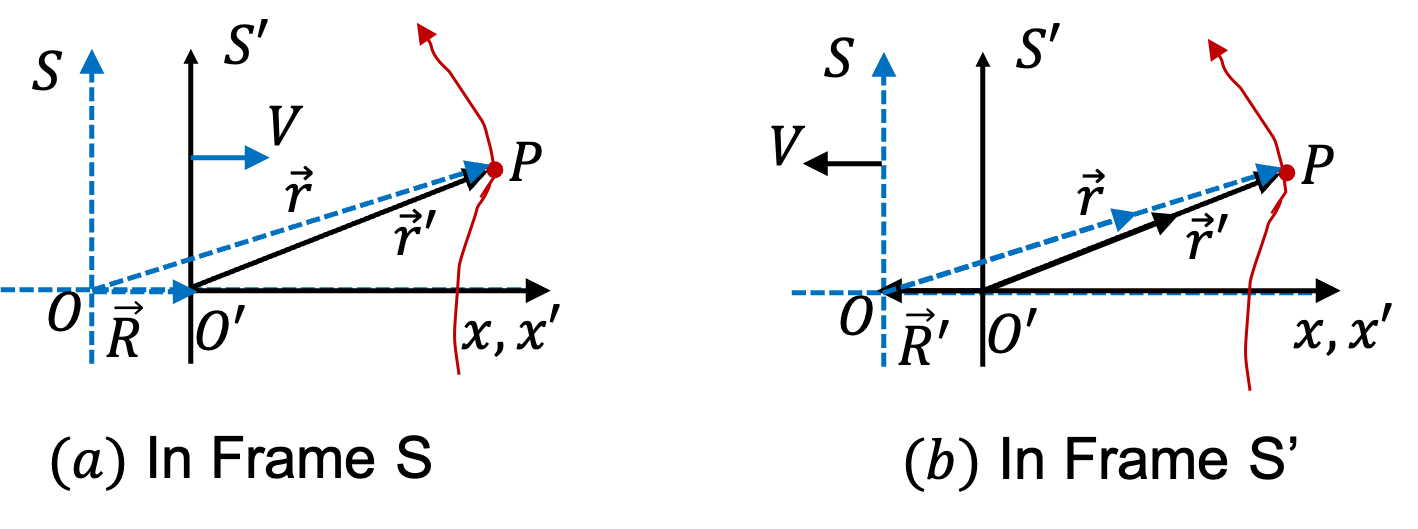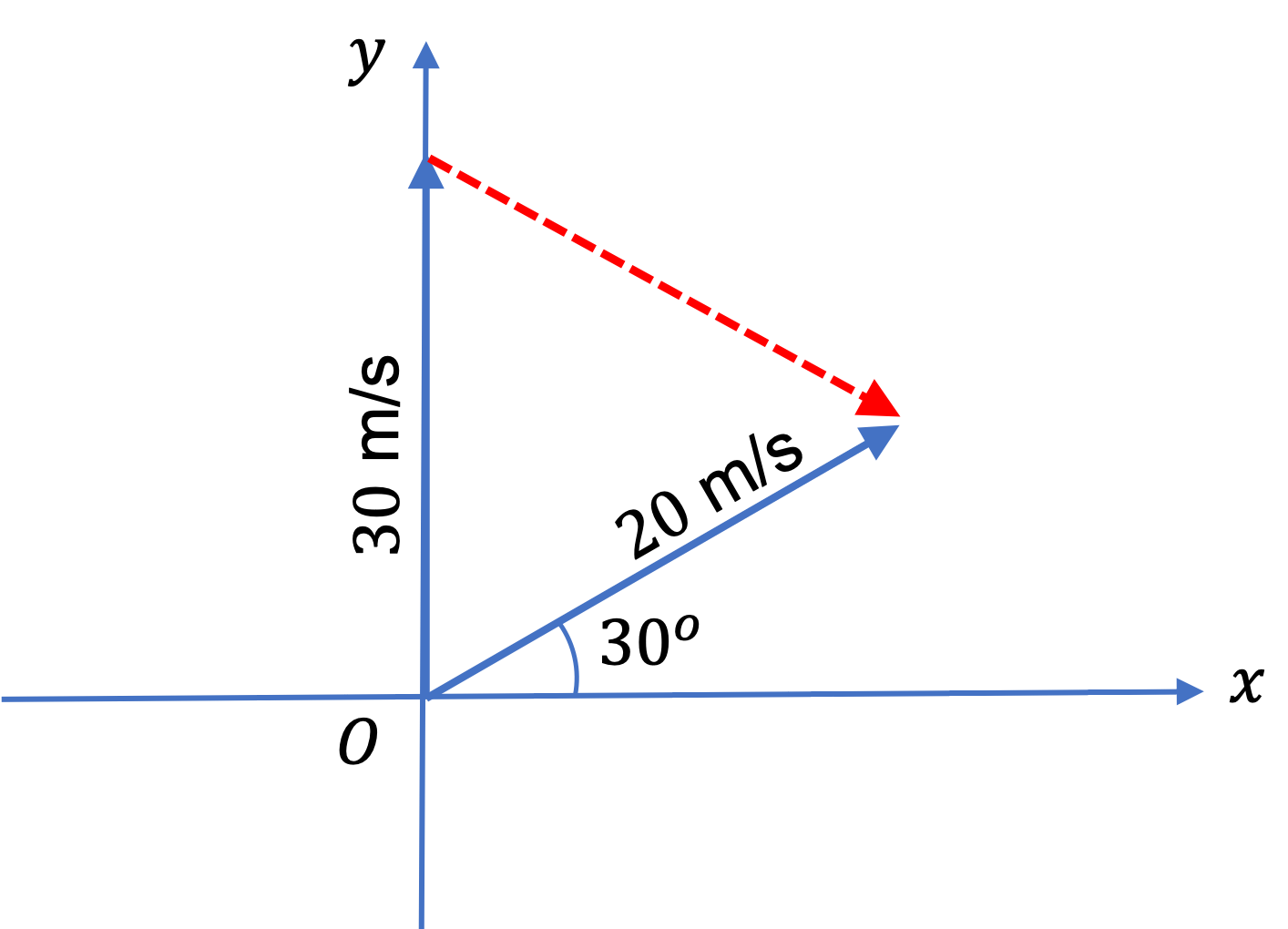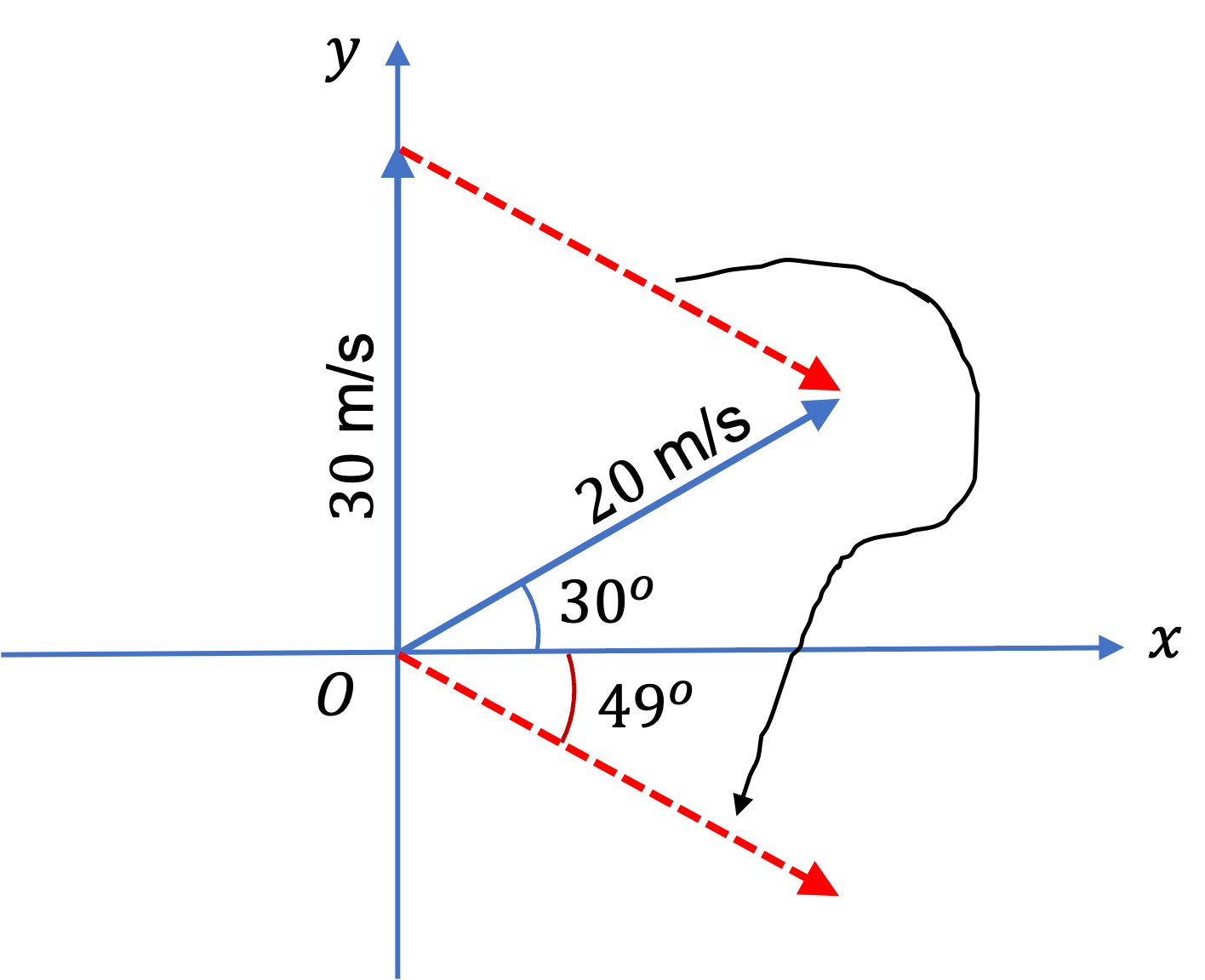## Section4.9Relative Motion in Different Frames

Have you ever wondered whether physics is same from the perspectives of two observers who are in a relative motion with respect to each other? For instance, what will be position, velocity and acceleration of the pendulum bob from observer S inside a moving train and observer S' on a fixed railway platform as in Figure 4.9.1? What is the relation between their values?

We will make one important assumption about time elapsed in the two frames. Let $\Delta t$ and $\Delta t'$ be values of time elapased between the same two events, e.g., time for a complete period. We will assume that

\begin{equation} \Delta t = \Delta t'.\tag{4.9.1} \end{equation}

This common-sense assumption about the relationship between time intervals in two reference frames that are in relative motion turns out to be incorrect as shown by Albert Einstein in $1905$ in his theory of special relativity. The mistake caused is ,however, very small for ordinary velocities, and becomes significant only when speeds are close to speed of light, which is $3\times10^8$ m/s.

Most situations encountered in everyday life have speeds that are much smaller than the speed of light, and hence we are on safe ground for most of our work. When we make this assumption, we say that we are in the realm of Galilean or non-relativistic relativity.

### Subsection4.9.1Observers Moving at Uniform Velocity With Respect to Each Other

To be concrete consider the description of motion of a particle P (say, the Bob of the pendulum) in frames S (say, the platform of railway station) and S' (say, the train) shown in Figure 4.9.2. Figure (a) on the left is drawn in frame S. In this frame origin O is not moving. The same physical sitution is dawn in (b), this time as viewd by frame S'; now, origin O' is not moving. We will use only Figure (a) to work out formulas that we need.Figure 4.9.2. The position of an object P with respect to two frames S and S'.

Frames S and S' have parallel Cartesian coordinates with motion of one frame with respect to the other happening only along common $x$ axis. For convenience, I will also set $t=t'=0$ at the instant the origins of the two frames coincide.

Let P be the location of the particle at instant $t=t\text{.}$ Let $\vec r$ denote its postion (vector) in S. Let $\vec r'$ denote the position in S'. Let $\vec R$ denote the postion of the origin of fame S' in frame S. These quantities are also given the following names.

1. $\vec r$ = Relative Position of P with respect to O.
2. $\vec r'$ = Relative Position of P with respect to O'.
3. $\vec R$ = Relative Position of O' with respect to O.

Let $V$ denote the relative speed of the two frames. As displayed in Figure 4.9.2(a), snce O' is moving with speed $V$ towards positive $x$ axis of S frame, we also have

\begin{equation} \vec R = V\; t\;\hat i,\label{eq-relative-origin-relation}\tag{4.9.2} \end{equation}

where $\hat i$ is the unit vector in the direction of positive $x$ axis. From the triangle $\triangle \text{OO'P}\text{,}$ I can immediately write the following.

\begin{equation} \vec r = \vec r' + \vec R.\label{eq-relative-postion-relation}\tag{4.9.3} \end{equation}

From these two equations, we get

\begin{equation*} \vec r = \vec r' + V\; t\;\hat i. \end{equation*}

We can decompose this into a relation among the Cartesian coordinates of P in the two frames.

\begin{align} \amp x = x' + V\; t\tag{4.9.4}\\ \amp y = y'\tag{4.9.5}\\ \amp z = z'\tag{4.9.6} \end{align}

Taking derivatives with respect to $t$ yields relations among the components of velocity of P.

\begin{align} \amp v_x = v'_x + V\tag{4.9.7}\\ \amp v_y = v'_y \tag{4.9.8}\\ \amp v_z = v'_z \tag{4.9.9} \end{align}

This says that speed in S frame will be different than speed in S' frame. In S frame speed is

\begin{align*} v_S \amp = \sqrt{v_x^2 + v_y^2 + v_z^2} \\ \amp = \sqrt{(v'_x+V)^2 + {v'_y}^2 + {v'_z}^2} \\ \amp = \sqrt{ v_{S'}^2 + V^2 + 2 v'_x V } \ne v_{S'} \end{align*}

The velocity vectors $\vec v = (v_x, v_y, v_z)$ and $\vec v' = (v'_x, v'_y, v'_z)$ are called relative velocity of P with respect to O and O', respectively. Taking derivative of velocity components gives relations among the components of acceleration of P. Since relative speed $V$ is constant, its derivative is zero. Hence, we get

\begin{align} \amp a_x = a'_x,\ \ (\text{since } \frac{dV}{dt} = 0.)\label{accelerations-in-twoframes-uniform-velocity}\tag{4.9.10}\\ \amp a_y =a'_y, \tag{4.9.11}\\ \amp a_z = a'_z. \tag{4.9.12} \end{align}

The acceleration vectors $\vec a = (a_x, a_y, a_z)$ and $\vec a' = (a'_x, a'_y, a'_z)$ are called acceleraions of P in S and S' respectively.

### Subsection4.9.2Observers Accelerating with Respect to Each Other

This is very common situation. For instance, you may want to describe motion of moon with respect to the Sun (say frame S) and with respect to the Earth (frame S'). Clearly S' is not moving about Sun with a uniform velocity. The question of observers accelerating with respect to each other in Figure 4.9.2 is simply the case of non-constant $V\text{,}$ that is

\begin{equation*} \frac{dV}{dt} = 0. \end{equation*}

Going over the calculations in section Subsection 4.9.1 above, you will note thatn everything is the same except Eq. (4.9.10). The one must be changed to

\begin{equation} a_x = a'_x + \frac{dV}{dt}.\tag{4.9.13} \end{equation}

Three runners A, B, and C are running on a straight East-West road. Runners A and B have velocities $\{ 5\ \text{m/s},\ \text{East}\}$ and $\{ 2\ \text{m/s},\ \text{West}\}\text{,}$ respectively, with respect to runner C. What is the relative velocity of the runner A with respect to the runner B?

Hint

Think: C as frame S, and B as frame S'. Also, work with vector components.

$7\text{ m/s}$ due East with respect to B.

Solution

We will think C as frame S, and B as frame S'. Then $\vec v_B$ will be $\vec V\text{,}$ the velocity of frame S' with respect to frame S, and we are after $\vec v'$ in formulas worked out in this section.

It is best to work analytically in a Cartesian coordinate system. Let the positive $x$-axis of the runner C be pointed towards East. Then, we can write the velocities of runners A and B in this frame.

\begin{align*} \amp \vec v_A = (5\ \text{m/s})\hat i, \\ \amp \vec v_B = (-2\ \text{m/s})\hat i. \end{align*}

The relative velocity of A with respect to B will be $\vec v_A - \vec v_B$ will be

\begin{equation*} \vec v_A - \vec v_B = (5\ \text{m/s})\hat i - (-2\ \text{m/s})\hat i = (7\ \text{m/s})\hat i. \end{equation*}

Therefore, the velocity of A is $7$ m/s towards the East with respect to B.

A train has velocity $20 \text{ m/s}$ in the direction $30^{\circ}$ North of East. A car has velocity $30 \text{ m/s}$ in the North direction. These velocities are given with respect to someone who is not moving with respect to the ground. What is the velocity of the train observed by the driver of the car? If you need to use a coordinate system, take your positive $x$ axis to point towards East and the positive $y$ to point towards North.

Hint

Think: Ground as frame S, Car as frame S', and train as P. Then, the problem is simply, given $\vec v$ and $>\vec V\text{,}$ what is $\vec v'\text{.}$

$26.5\text{ m/s}\text{,}$ $49^{\circ}$ South of East.

Solution

We will think the Ground as frame S, Car as frame S', and train as P. Also, take East as $x$-axia and North as $y$-axis. This gives the following for velocities of train and car in frame S.

\begin{align*} \amp \vec v_t = \left( 20 \cos\, 30^{\circ}, 20 \sin\, 30^{\circ}\right) = (17.32, 10)\\ \amp \vec V_c = \left( 0, 30 \right) \end{align*}

Let $\vec v^{\prime}_t$ be the velocity of the train with respect to the car. We have the following vector relations.

\begin{align*} v^{\prime}_t \amp = \vec v_t - \vec V_c\\ \amp = (17.32-0, 10-30) = (17.32, -20). \end{align*}Therefore, if you look at the train from the car you will notice it is moving with speed $\sqrt{17.32^2 + 20^2}\text{ m/s} = 26.5\text{ m/s}$ in the direction $49^{\circ}$ South of East, i.e., clockwise (since $\arctan(y/x)$ results in a negative value) from positive $x$ axis into the fourth quadrant.Suppose you want to cross a river by a boat. Assume river is flowing at a speed of $2\text{ m/s}$ at all points across the river. Let speed of the boat in calm waters be $5\text{ m/s}\text{.}$ If river was not flowing much, assuming static, you will just point the boat to the exact opposite point of the shore and you will get there in $30\text{ sec}\text{.}$

But to cross the raging river you need to point the boat in the direction up the river. That is, to get your net velocity to point across the river, you want velocity of the boat with respect to the river be pointed in the direction up the river.

(a) Find angle $\theta$ you need. (b) Find the time it will take you to cross the river.

Hint

Note: Speed with respect to calm waters means it is with respect to River frame. Take Shore as frame S and River as frame S'.

Solution

Here boat is P, shore is frame S and river is frame S'. From the data of crossing of river in calm waters we get speed $v'_B$ in the figure, which stands for the speed of the boat with respect to the River frame.

\begin{equation*} v' \equiv v'_B = 5\text{ m/s}. \end{equation*}

We also know the speed of frame S' with respect to S.

\begin{equation*} V \equiv = v_R = 2\text{ m/s} \end{equation*}

We want to find the value of speed $v \equiv v_B$ of the boat with respet to S. From the triangle of vectors in Figure 4.9.6 we get the following vector equation.

\begin{equation*} \vec v_B = \vec v'_B + \vec v_R. \end{equation*}

This equation has the following $x$ and $y$ components.

\begin{align*} \amp 0 = -v'_B\sin\theta + v_R \\ \amp v_B = v'_B\cos\theta + 0 \end{align*}

From the first equation, we will get $\theta\text{,}$ which we will use in the second to get $v_B\text{.}$

\begin{align*} \amp \theta = \cos^{-1}(v_R/v'_B) = \sin^{-1}(2/5) =23.6^\circ.\\ \amp v_B = v'_B\cos\theta = 4.6\text{ m/s}. \end{align*}

From the problem description, we know the width of the river to be

\begin{equation*} w = 5\text{m/s} \times 30\text{ s} = 150\text{ m}. \end{equation*}

Therefore, the time for crossing now will be

\begin{equation*} t = \frac{w}{v_B} = \frac{150}{4.6} = 32.6\text{ m/s}. \end{equation*}

Try Checkpoint 4.9.5 one more time, but now, for a landing site on the opposite bank that is $50\text{ m}$ upstream from exact opposite point.

Solution

No solution provided.

A marble is dropped in an elevator that is accelerating at $3\ \text{m/s}^2$ upward with respect to the ground. Find the acceleration of the marble with respect to the ground and with respect to an observer in the elevator.Hint

Set up the equation for vertical components of acceleration vector relation.

$9.81\text{ m/s}^2$ and $12.81\text{ m/s}^2$

Solution

We draw a picture of the situation given in the problem in Figure 4.9.9 where we denote the ground-based observer as $O$ and the observer in the elevator as $O'\text{.}$ The ball is falling freely with $g$ as far as the ground-based observer is concerned. Therefore, the magnitude of $a = g = 9.81\text{ m/s}^2\text{.}$ With $\hat j$ pointed up, we have

\begin{equation*} \vec a = - 9.81\,\hat j, \ \ \vec A = 3\,\hat j, \ \ \vec a^{\prime} = a^{\prime}\, \hat j. \end{equation*}

The accelerations of the marble with respect to the observers $O\text{,}$ and $O'$ are related as follows.

\begin{equation*} \vec a = \vec A + \vec a^{\prime}. \end{equation*}

Therefore,

\begin{equation*} - 9.81 = 3 + a', \end{equation*}

which give the following value for the $y$-component of the acceleration of the marble for the observer in the elevator.

\begin{equation*} a' = -12.81\ m/s^2. \end{equation*}

Therefore, the acceleration of the marble will be $-12.8\ \text{m/s}^2$ in the elevator frame, regardless of whether the elevator is moving up or down and the value of the speed of the elevator as long as the acceleration of the elevator with respect to ground is $3\ \text{m/s}^2$ pointed up.

If you were in this elevator and standing on a balance, you would notice that your “weight” is more than when the elevator was not accelerating up. We say that $ma'$ is the apparent weight.

(a) Try Checkpoint 4.9.8 one more time, but now, let the accelerator be going up and slowing down with magnitude of deceleration being $3\text{ m/s}^2\text{.}$ (b) What will happen if the elevator is going down and accelerating at $3\text{ m/s}^2\text{?}$ You would be wrong if you got the same answer as in Checkpoint 4.9.8.

Solution

No solution provided.

Suppose raindrops are falling vertically with some speed $v$ as viewed by an stationary observer on the ground. When he starts to run, he notices the rains make an angle when they hit his face. The faster he runs more the angle. When he runs at speed $V\text{,}$ angle is $\theta\text{.}$ Find a relation between $v\text{,}$ $V\text{,}$ and $\theta\text{.}$

Hint: Think Ground as frame S, runner as frame S'

Solution

No solution provided.